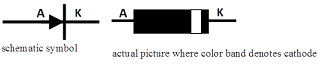## Diode

A semiconductor diode is an electronic component consists of two electrodes, the anode and the cathode. The anode (A) is the positive electrode of diode while cathode (K) is the negative electrode. The anode allows only positive charges and blocks negative charges while cathode allows negative charges and blocks positive charges. The diode acts as one-way valve for the flow of current. This means that the diode allows current to follow through it in one direction and blocks its flow in the other direction.Semiconductor Diode (Schematic symbol)

There are two methods of biasing diodes; the forward bias and reverse bias. A diode is forward-biased if its anode is connected to the positive terminal of the source and reverse-biased if the cathode is connected to the negative. In forward bias, the diode acts like a closed switch where current passes through it easily; while in reverse bias the diode acts like a switch that is open where it almost stops the current flow.Biasing Diode

The voltage dropped across a forward-biased diode is called the forward voltage. The forward voltage of a silicon diode is approximately 0.7 volts while the germanium diode has 0.3 volts. The maximum reverse bias voltage that a diode can handle without breaking it down is called Peak Inverse Voltage, or PIV rating.

There are several types of diodes: the rectifier diode, zener, light emitting diode, varactor, photodiode and more.

#### Zener diode

is designed to limit the voltage across its terminal in reverse bias. This is the reason why zener diode is usually used as voltage regulator.

#### Varactor diode

also called as variable-capacitance diode is commonly used in electronic tuning circuits.

#### Light emitting diode (LED)

is a diode that emits light when properly biased.

#### Photodiode

operates in reverse bias and has a small transparent window which allows light to strike the PN junction.Some Types of Diodes and corresponding symbols (Rectifier, Zener, Varactor, LED, Photodiode)